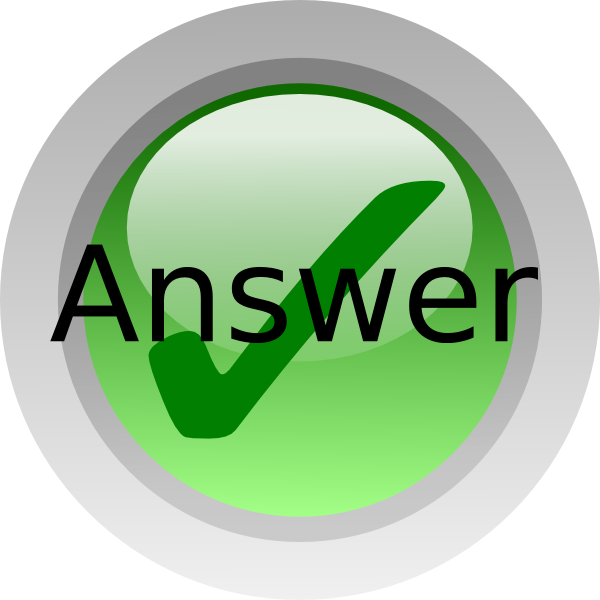SYMBOLS WE USE TO SOLVE OUR ANSWERS IN MATHS AND OTHERS | EarlyAnswer, Best Waec, Neco Expo Site Runs

###ONLINE CANDIDATES : 31### Confirmation Page# SYMBOLS WE USE TO SOLVE OUR ANSWERS IN MATHS AND OTHERS

SYMBOL CLASS FOR MATHS,PHYSICS, CHEMISTRY, ACCOUNT,

This is how we send our Maths Symbols and the way you are suppose to write it on ur paper.

SCROLL DOWN TO VIEW MORE

(1) / means division or divide
Examples:
2/2 means 2/2
2 whole no 3/4 means 2¾
M=wp+(1/3)yp^2 means m=wp + ¾y2
(2) Log means logarithm
Example: (8) Log5 (base 8) means Log85
(3) * means multiplication
Example: 2*2 means 2×2
(4) ^ means Raise to power
Examples:
3^(-1) means 3-1
5^(2) means 52
30cm^(-2) means 30cm-2
(5) Tita means θ
Example: Tan tita means tan θ
(6) Pie means π
Example: Pie R sqr h means πr2h
(7) Base means subscript
Example: 2 Base 3 means A3
(8) Sqr root means √
Examples:
√3-√6+2√3 means Root 3-root6+2root 3
√(¾) means sqr root(3/4)
(9) bar means a dash on top a number of letter
Example: X bar means x̅
(10) —(1) means equation 1
(11) Proportional means ∝
Example: R is proportional to 3/4rut m means R ∝ ¾
(12) Alpha means α
(13) Beta means β
(14) Gamma means γ
(15) cube root means ∛
(16) Mu means μ
(17) Rho means ρ
(18) Delta means δ
(19) Sigma means σ
(20) Tau means τ
(21) Ohm means Ω
(22) Lamda means λ
(23) Omega means ω
(24) Intersection means ∩
Example: B intersection C means B∩C
(25) Union means U
Example: B union C means BUC
(26) Factorial means !
(27) complements in sets means ‘
(28) (aq),(g),(l),(s) is used in chemistry to show the state of matter in equations
(29) Equivalent to means ≡
(30) Not equal to means ≠
(31) Quotation means “ ”
(32) Less or equal to means ≤
(33) Greater or equal to ≥
Example: (3/4)>=(1/2) means ¾ ≥ ½
(34) Plus or minus (or +-) means ±
Example: (3/4)<=(1/2) means ¾ ≤ ½
(35) Element of means ∈
Example: 2 belongs to X or 2 is a member of X means 2∈X
(36) Percent or percentage means %
(37) Degrees Fahrenheit means ℉
(38) Degrees Celsius means ℃
(39) Universal set means ξ or U
(40) Null or empty set means ∅ or {}
(41) <RST means RŜT
(42) 2.S.F means 2 significant figures
(43) P=(2,3) means P={2,3} in sets
(44) Propotional to means ∝
(45) Therefore means ∴
(46) KNO(3)(s) means KNO3(s)
(47) NaCl=> Na^(+) + Cl^(-) means NaCl → Na++ Cl
(48) Summation means Ʃ
(49) Parallel lines means ǁ
(50) Perpendicular lines means ˩
(51) Reversible equation means ↔
(52) Infinity means ∞
(53) Approximately means ~
(57) Approximately equal to ≅

CHECK OUT:  2020 WAEC MATHEMATICS OBJ AND ESSAY QUESTIONS/ANSWERS VERIFIED EXPO

Other Symbols
(1) File means ɸ
(2) Δ means Change or Triangle
(3) slope or differentiation means dy/dx or ∂y/∂x or δy/δx or Δy/Δx
(4) Parenthesis means …
(5) Naira means ₦
(6) Integral means ∫
(7) Almost equal to means ≈
(8) For all means ∀
(9) There exist means ∃
(10) Epsilon means ε
(11) Vertical dots means ⁞Join Our Telegram Channel |Whatsapp Our Admins: Click Any Name Mr.Paul or Mr.Henry

### Comment

Posted by on 27th April 2019.

Categories: WAEC

## 4 Responses

1. pls after sending the card for english language can u send the answer through message to my phone?

by salami afeez on May 4, 2019

2. We send answers to whatsapp only

by admin on May 4, 2019

3. Sir what of we that have subscribed for economic ate we going to use same pin

by Sir what of we that have subscribed for economic ate we going to use same pin on Jul 5, 2019

4. Yes, use the same pin

by admin on Jul 5, 2019